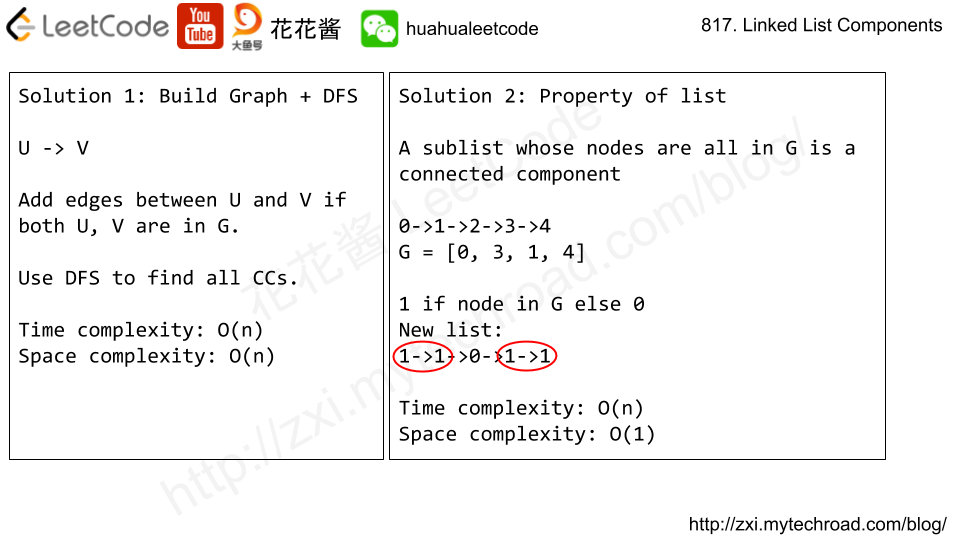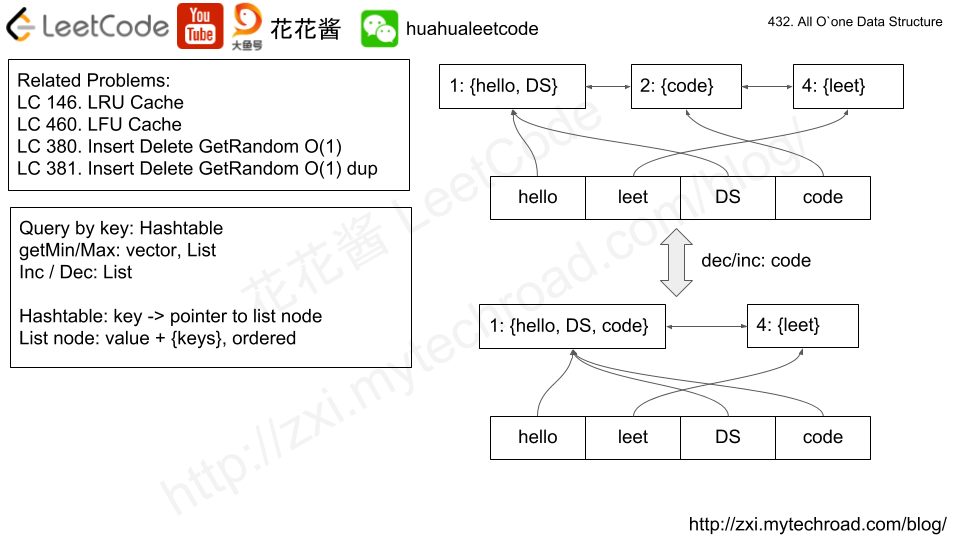# Problem

You are given two non-empty linked lists representing two non-negative integers. The most significant digit comes first and each of their nodes contain a single digit. Add the two numbers and return it as a linked list.

You may assume the two numbers do not contain any leading zero, except the number 0 itself.

What if you cannot modify the input lists? In other words, reversing the lists is not allowed.

Example:

Input: (7 -> 2 -> 4 -> 3) + (5 -> 6 -> 4)
Output: 7 -> 8 -> 0 -> 7

# Solution: Simulation

Using a stack to “reverse” the list. Simulate the addition digit by digit.

Time complexity: O(l1 + l2)

Space complexity: O(max(l1, l2))

C++

# Problem

We are given head, the head node of a linked list containing unique integer values.

We are also given the list G, a subset of the values in the linked list.

Return the number of connected components in G, where two values are connected if they appear consecutively in the linked list.

Example 1:

Input:
G = [0, 1, 3]
Output: 2
Explanation:
0 and 1 are connected, so [0, 1] and  are the two connected components.


Example 2:

Input:
G = [0, 3, 1, 4]
Output: 2
Explanation:
0 and 1 are connected, 3 and 4 are connected, so [0, 1] and [3, 4] are the two connected components.


Note:

• If N is the length of the linked list given by head1 <= N <= 10000.
• The value of each node in the linked list will be in the range [0, N - 1].
• 1 <= G.length <= 10000.
• G is a subset of all values in the linked list.# Problem

Given a singly linked list, group all odd nodes together followed by the even nodes. Please note here we are talking about the node number and not the value in the nodes.

You should try to do it in place. The program should run in O(1) space complexity and O(nodes) time complexity.

Example:
Given 1->2->3->4->5->NULL,
return 1->3->5->2->4->NULL.

Note:
The relative order inside both the even and odd groups should remain as it was in the input.
The first node is considered odd, the second node even and so on …

Credits:
Special thanks to @DjangoUnchained for adding this problem and creating all test cases.

# Solution

Time complexity: O(n)

Space complexity: O(1)

C++

V2

Java

Python3

# Problem

https://leetcode.com/problems/all-oone-data-structure/description/

Implement a data structure supporting the following operations:

1. Inc(Key) – Inserts a new key with value 1. Or increments an existing key by 1. Key is guaranteed to be a non-empty string.
2. Dec(Key) – If Key’s value is 1, remove it from the data structure. Otherwise decrements an existing key by 1. If the key does not exist, this function does nothing. Key is guaranteed to be a non-empty string.
3. GetMaxKey() – Returns one of the keys with maximal value. If no element exists, return an empty string "".
4. GetMinKey() – Returns one of the keys with minimal value. If no element exists, return an empty string "".

Challenge: Perform all these in O(1) time complexity.# Solution

Time complexity: O(1)

Space complexity: O(n), n = # of unique keys

# Problem:

Given a singly linked list, return a random node’s value from the linked list. Each node must have the same probability of being chosen.

What if the linked list is extremely large and its length is unknown to you? Could you solve this efficiently without using extra space?

Example:

# Solution:

C++

Time Complexity: O(n)

Space Complexity: O(1)

Mission News Theme by Compete Themes.